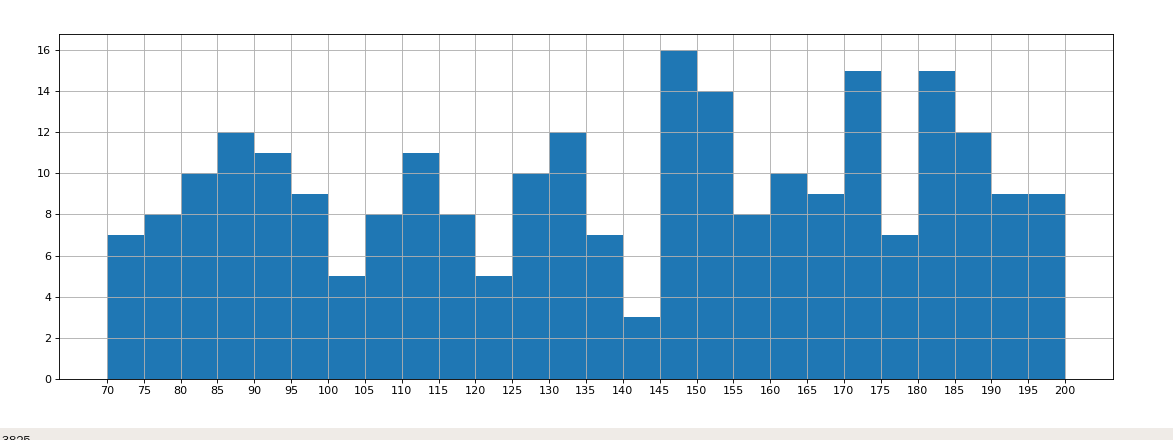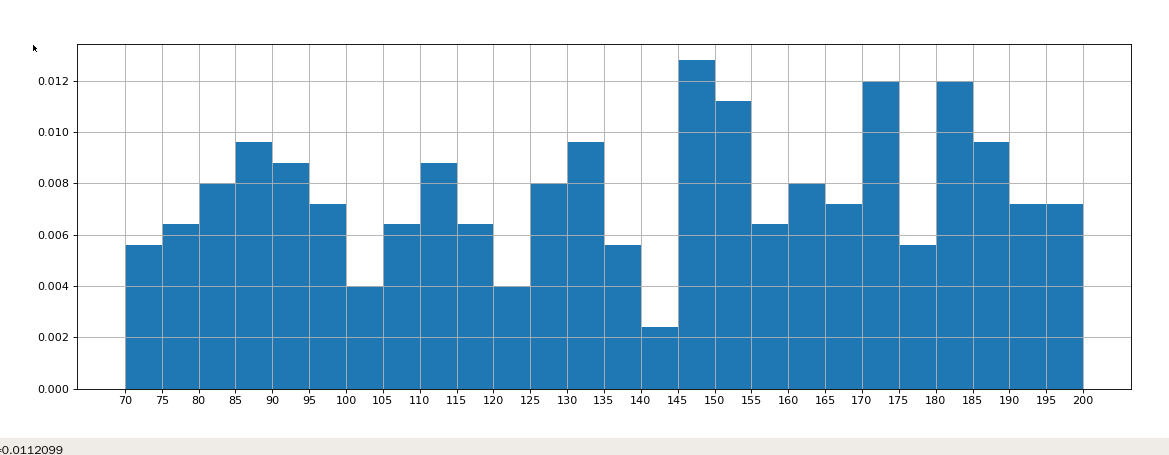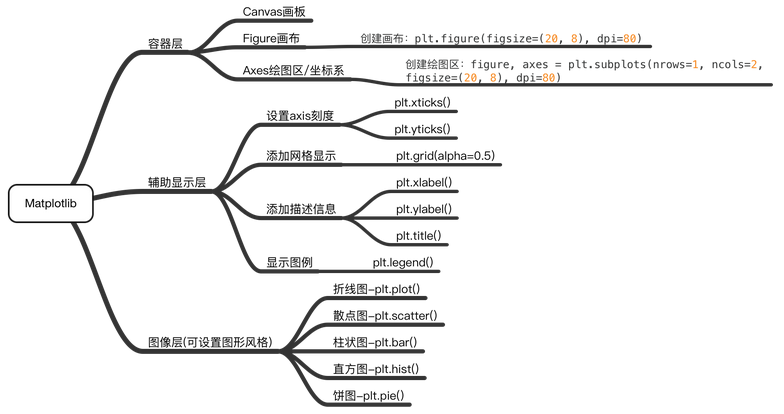• 方法：将共有30名参与者（均患有PFPS的所有女篮球运动员，平均年龄为20-30岁）分为两个等/等渗和对照实验。 建议参加者进行为期八周的等和等渗锻炼，重点是在两个四个星期的阶段中进行的下肢EMSII锻炼。 ...
• 结果表明齿状和无牙在下颌管长度（p = 0.030），牙槽al的距离（p <0.001）和颊皮质骨的距离（p <0.001）之间存在显着差异，其中齿状值明显更高耐心。 至于性别，女性的神经丛和牙槽骨c之间的距离（p &...
• 组距：指每个小组的两个端点的距离，公式如下： 组数=极差/组距 应用场景 用户的年龄分布状态 一段时间内用户的点击次数的分布状态 用户活跃时间的分布状态 案例 现有250个数据，分别是电影的时间长度，统计...
把数据分成多少组进行统计

注意：组数要适当，太少会有较大的统计误差，太多规律不明显

组数：将数据分组，当数据在100个以内时，按数据多少需分5-12组

组距：指每个小组的两个端点的距离，公式如下：

组数=极差/组距

应用场景

用户的年龄分布状态
一段时间内用户的点击次数的分布状态
用户活跃时间的分布状态
案例

现有250个数据，分别是电影的时间长度，统计每个时间段内的电影数或出现的频率


# import random

# print([random.randint(70, 200) for i in range(250)])

from matplotlib import pyplot as plt
from matplotlib import font_manager

my_font = font_manager.FontProperties(fname='/usr/share/fonts/cjkuni-uming/uming.ttc')

a = [154, 191, 101, 153, 95, 118, 184, 98, 159, 120, 191, 159, 115, 172, 131, 172, 191, 102, 180, 139, 189, 170, 174,
188, 111, 132, 148, 74, 195, 151, 92, 181, 172, 124, 149, 151, 172, 139, 111, 124, 152, 167, 167, 185, 195, 170,
172, 147, 175, 154, 151, 182, 169, 91, 136, 113, 112, 70, 153, 82, 148, 110, 178, 194, 87, 133, 148, 180, 151,
173, 127, 148, 186, 197, 162, 138, 196, 150, 103, 76, 130, 78, 71, 128, 187, 91, 90, 161, 72, 112, 98, 190, 93,
182, 182, 93, 150, 138, 76, 135, 187, 196, 169, 134, 129, 151, 146, 109, 152, 88, 119, 100, 120, 122, 119, 182,
95, 183, 110, 181, 81, 160, 138, 89, 97, 166, 182, 127, 108, 87, 158, 73, 88, 162, 105, 128, 79, 79, 193, 162,
181, 128, 130, 145, 129, 111, 87, 169, 87, 105, 86, 92, 149, 80, 106, 198, 188, 140, 179, 149, 125, 163, 95, 131,
185, 187, 143, 82, 193, 148, 157, 179, 146, 107, 82, 87, 75, 98, 75, 112, 102, 163, 152, 112, 160, 129, 84, 186,
161, 200, 196, 93, 141, 86, 95, 83, 93, 112, 127, 149, 109, 145, 92, 130, 195, 85, 178, 175, 194, 170, 170, 177,
180, 158, 148, 93, 190, 134, 72, 158, 156, 180, 146, 80, 156, 86, 70, 163, 172, 185, 183, 77, 132, 107, 167, 173,
134, 130, 167, 96, 118, 189, 82, 170, 118, 119, 168, 82, 174, 119]

plt.figure(figsize=(20, 8), dpi=80)

# 计算组数
d = 5  # 组距
num_bins = (max(a)-min(a))//d

plt.hist(a, num_bins)

# 设置x轴的刻度
plt.xticks(range(min(a), max(a)+d, d))

plt.grid()

plt.show()如果计算出现的频率，只需改动一处

plt.hist(a, num_bins, normed=True)展开全文• 直方图更多的应用场景: 用户年龄的分布状态; 一段时间内用户的点击数分布状态; 用户活跃时间的分布状态; ...哪些数据可以绘制直方图?...组距: 每个小组里面端点的距离; 组数 = 极差 / 组距 ...


直方图更多的应用场景:

用户年龄的分布状态;
一段时间内用户的点击数分布状态;
用户活跃时间的分布状态;

哪些数据可以绘制直方图?

连续的数据;
没有统计过的数据;
原始数据;

案例1: 250部电影的时长, 电影时长的分布状态;

把数据分为多少组进行统计?

如果数据在100个以内, 一般分为5-12组;
组距: 每个小组里面端点的距离;
组数 = 极差 / 组距

import random
from matplotlib import  pyplot as plt

y = [random.randint(60, 180) for i in range(250)]

# 组距
d = 10

# 组数
num_bins = (max(y) - min(y)) // d

# 设置x轴的刻度范围
plt.xticks(list(range(min(y), max(y) + d))[::d])

# 设置网格
plt.grid(linestyle='-.', alpha=0.3)

# 直方图绘制数据分为20个分组
plt.hist(y, num_bins)
plt.savefig('doc/01_hist.png')


案例2: 美国人从家到上班地点所需时间的统计

数据来源: https://en.wikipedia.org/wiki/Histogram#Examples
Data by absolute numbers

Interval  Width	   Quantity	 Quantity/width
0			5		4180		836
5			5		13687		2737
10			5		18618		3723
15			5		19634		3926
20			5		17981		3596
25			5		7190		1438
30			5		16369		3273
35			5		3212		642
40			5		4122		824
45			15		9200		613
60			30		6461		215
90			60		3435		57

from matplotlib import pyplot as plt

# 公司到家的的距离
interval = [0, 5, 10, 15, 20, 25, 30, 35, 40, 45, 60, 90]
# 间距
width = [5, 5, 5, 5, 5, 5, 5, 5, 15, 30, 60]
# 对应的人数
quantity = [836, 2737, 3723, 3926, 3596, 1438, 3273, 642, 824, 613, 215, 57]
# 设置图形的大小
plt.figure(figsize=(10, 10))
# 绘制条形图
# plt.bar(range(12), quantity, width=1)
# 绘制折线图
plt.plot(interval, quantity)
# 设置x轴的刻度
# _x = [i-0.5 for i in range(12)]
# plt.xticks(_x, labels=interval)
plt.xticks(interval)
# 设置网格
plt.grid(linestyle='-.', alpha=0.5)
# gca====get current axis 获取当前的坐标轴
ax = plt.gca()
# 设置右边框和上边框;
ax.spines['right'].set_color('none')
ax.spines['top'].set_color('none')
# 设置x轴为下边框
ax.xaxis.set_ticks_position('bottom')
# 设置y轴为作边框
ax.yaxis.set_ticks_position('left')
# 设置x轴和y轴的交点为(0, 0)点;
ax.spines['bottom'].set_position(('data', 0))
ax.spines['left'].set_position(('data', 0 ))

# 保存图片
plt.savefig('doc/03_bar.png')



展开全文matplotlib python
• 上期中介绍了两种利用非线性函数拟合人口与物种增长趋势的方法。这两种方法都可以用于对人口与物种增长的总体趋势进行预测，但预测不够精细。...我们把整个社会中的人群按年龄分成n，每中该年的人口总数...
上期中介绍了两种利用非线性函数拟合人口与物种增长趋势的方法。这两种方法都可以用于对人口与物种增长的总体趋势进行预测，但预测不够精细。我们知道在正常社会条件或自然条件下，生育率与死亡率是与群体的年龄构成息息相关的。我们需要对整个群体按年龄进行层次划分，构建与年龄相联系的人口模型。典型的例子就是Leslie矩阵模型。
Leslie矩阵介绍
我们把整个社会中的人群按年龄等距分成n组，每组中该年的人口总数为$a_i,i=1,2,...,n$，每组人口的每年的普遍存活率为$c_i,i=1,2,...,n-1$(设最后一组下一年全部死亡)，每组人口的每年普遍生育率为$b_i,i=1,2,...,n$，则下一年每组中的人口总数$a'_i,i=1,2,...,n$就满足递推关系式$\begin{cases}a'_i=a_{i-1}c_{i-1},i=2,3,...,n\\a'_1=\sum_{i=1}^{n}a_ib_i\end{cases}$
该式可写成矩阵乘向量的形式：
$\vec{a'}= \left( \begin{matrix} b_1&b_2&...&b_{n-1}&b_n\\ c_1&0&...&0&0\\ 0&c_2&...&0&0\\ \vdots&\vdots&&\vdots\\ 0&0&...&c_{n-1}&0 \end{matrix} \right) (a_1,a_2,...,a_n)^T$
该式中左边的矩阵就是Leslie矩阵。
Leslie矩阵性质

Leslie矩阵有唯一的单重正特征值$\lambda_1$，对应的特征向量$\vec x_1=(1,b_1/\lambda_1,c_1c_2/\lambda_1^2,...,c_1c_2...c_{n-1}/\lambda_1^{n-1})^T$

证明：设n阶的该矩阵为Ln，n阶的特征多项式为Pn，则有
$P_n=|\lambda I-L_n|= \left| \begin{matrix} \lambda-b_1&-b_2&...&-b_{n-1}&-b_n\\ -c_1&\lambda&...&0&0\\ 0&-c_2&...&0&0\\ \vdots&\vdots&&\vdots&&\\ 0&0&...&-c_{n-1}&\lambda \end{matrix} \right|$
$=>P_n=\lambda \left| \begin{matrix} \lambda-b_1&-b_2&...&-b_{n-2}&-b_{n-1}\\ -c_1&\lambda&...&0&0\\ 0&-c_2&...&0&0\\ \vdots&\vdots&&\vdots&&\\ 0&0&...&-c_{n-2}&\lambda \end{matrix} \right|+c_{n-1} \left| \begin{matrix} \lambda-b_1&-b_2&...&-b_{n-3}&-b_{n-1}\\ -c_1&\lambda&...&0&0\\ 0&-c_2&...&0&0\\ \vdots&\vdots&&\vdots&&\\ 0&0&...&-c_{n-2}&0 \end{matrix} \right|$
$=>P_n=\lambda P_{n-1}+c_{n-1}(-b_{n-1})(c_1c_2...c_{n-2})(-1)^{n-2}(-1)^{n-2}=> P_n=\lambda P_{n-1}-b_{n-1}c_1c_2...c_{n-1}$
$=>P_n=\lambda P_{n-1}-\beta_{n-1}=> P_n=\lambda^n-\beta_1\lambda^{n-1}-\beta_2\lambda^{n-2}-...-\beta_n=>$
$0=\lambda^n-\beta_1\lambda^{n-1}-\beta_2\lambda^{n-2}-...-\beta_n=>P_n=\lambda^n-\beta_1\lambda^{n-1}-\beta_2\lambda^{n-2}-...-\beta_n=>$
$1=\beta_1\lambda^{-1}+\beta_2\lambda^{-2}+...+\beta_n\lambda^{-n}$右边的函数是单调连续减函数，且$\lambda$无穷大时趋近0、$\lambda$趋近于0时趋近正无穷，所以有唯一正特征根$\lambda_1$，对应的特征向量为$\vec x_1=(1,b_1/\lambda_1,c_1c_2/\lambda_1^2,...,c_1c_2...c_{n-1}/\lambda_1^{n-1})^T$

所有负的特征值都满足$|\lambda|<\lambda_1$，称$\lambda_1$为严格优势特征值

证明:设有特征值满足$|\lambda|\geq\lambda_1=>\lambda\geq-\lambda_1$，则有其依然满足$1=\beta_1\lambda^{-1}+\beta_2\lambda^{-2}+...+\beta_n\lambda^{-n}$ ，而 $1=\beta_1\lambda_1^{-1}+\beta_2\lambda_1^{-2}+...+\beta_n\lambda_1^{-n} \geq\beta|\lambda^{-1}|+\beta|\lambda^{-2}|+...+\beta|\lambda^{-n}|>\beta\lambda^{-1}+\beta\lambda^{-2}+...+\beta\lambda^{-n}$，矛盾

对于任意人口分布向量$\vec x$，其迭代k次后的结果有$\displaystyle \lim_{k->+∞} \frac{\vec x^{(k)}}{\lambda_1^k}=c\vec x_1$(c为常数)，即迭代了无穷多次时，人口的分布比例趋近于特征向量$\vec x_1$，而人口增长率趋近于特征值$\lambda_1$

证明：仅对可化为对角阵的情况进行证明（一般情况需要用到约旦标准型）。$\displaystyle \lim_{k->+∞} \frac{\vec x^{(k)}}{\lambda_1^k}=\displaystyle \lim_{k->+∞} \frac{L^k\vec x^{(0)}}{\lambda_1^k}=\displaystyle \lim_{k->+∞} \frac{(Pdiag(\lambda_1,\lambda_2,...,\lambda_n)P^{-1})^k\vec x^{(0)}}{\lambda_1^k}=\displaystyle \lim_{k->+∞} \frac{Pdiag(\lambda_1^k,\lambda_2^k,...,\lambda_n^k)P^{-1}\vec x^{(0)}}{\lambda_1^k}=\displaystyle \lim_{k->+∞} Pdiag(1,\lambda_2^k/\lambda_1^k,...,\lambda_n^k/\lambda_1^k)P^{-1}\vec x^{(0)}$，由于$\lambda_1$为严格优势特征值，有$原式=\displaystyle \lim_{k->+∞} Pdiag(1,0,...,0)P^{-1}\vec x^{(0)}=(\vec x_1,\vec x_2,...,\vec x_n)diag(1,0,...,0)(\vec x'_1,\vec x'_2,...,\vec x'_n)(a_1,a_2,...,a_n)^T=c\vec x_1$
总结
列出Leslie矩阵，我们即可对人口年龄分布进行迭代。且无论一开始的人口分布向量如何，人口比例在迭代无数次之后总趋近于特征向量$\vec x_1$。而人口增长率趋近于特征值$\lambda_1$，说明特征值$\lambda_1$可以用于预测人口增长速度，对于计生有重要意义。


展开全文线性代数 人口模型
• 组距式分组;二单项式数组的频数分析;1对年龄按升序排序 2将各组的年龄值输入到工作表中作为组界 3单击插入单击函数选择FREQUENCY 4选择组界区域和数据区域的单元格地址 单击确定 5按下F2+Ctrl+Shift+Enter得到频数...
• 直方图描述的是一组数据的频次分布，是以矩形的长度表示每一组的频数或数量，宽度则表示各组的组距，因此其高度与宽度均有意义，利于展示大量数据集的统计结果。例如把年龄分成“0-5，5-10，……，80-85”17个组，...
直方图

直方图与柱状图的对比

柱状图是以矩形的长度表示每一组的频数或数量，其宽度(表示类别)则是固定的，利于较小的数据集分析。

直方图描述的是一组数据的频次分布，是以矩形的长度表示每一组的频数或数量，宽度则表示各组的组距，因此其高度与宽度均有意义，利于展示大量数据集的统计结果。例如把年龄分成“0-5，5-10，……，80-85”17个组，统计一下中国人口年龄的分布情况。直方图有助于我们知道数据的分布情况，诸如众数、中位数的大致位置、数据是否存在缺口或者异常值。

1. 直方图展示数据的分布，柱状图比较数据的大小。

​ 这是直方图与柱状图最根本的区别。举个例子，有10个苹果，每个苹果重量不同。如果使用直方图，就展示了重量在0-10g的苹果有多少个，10-20g的苹果有多少个；如果使用柱状图，则展示每个苹果的具体重量。

所以直方图展示的是一组数据中，在你划分的区间里，这些数据的分布情况，但是我们不知道在一个区间里，单个数据的具体大小。上图展现了游客在博物馆的游览时间，其中，将近40％的游客仅逗留了0-10分钟。但是我们无法知道这些游客中，每个人具体的游览时间是多少。

​ 而在柱状图里，我们能看到的是每个数据的大小，并且进行比较。下图就比较了在12次展览中，参观者参观时间的中位数，我们能够知道参观的具体用时。

2. 直方图X轴为定量数据，柱状图X轴为分类数据。

​ 在直方图中，X轴上的变量是一个个连续的区间，这些区间通常表现为数字，例如代表苹果重量的“0-10g，10-20g……”，代表时间长度的“0-10min，10-20min……”。而在柱状图中，X轴上的变量是一个个分类数据，例如不同的国家名称、不同的游戏类型。

​ 直方图上的每根柱子都是不可移动的，X轴上的区间是连续的、固定的。而柱状图上的每根柱子是可以随意排序的，有的情况下需要按照分类数据的名称排列，有的则需要按照数值的大小排列。

3. 直方图柱子无间隔，柱状图柱子有间隔

因为直方图中的区间是连续的，因此柱子之间不存在间隙。而柱状图的柱子之间是存在间隔。

4. 直方图柱子宽度可不一，柱状图柱子宽度须一致

​ 柱状图柱子的宽度因为没有数值含义，所以宽度必须一致。但是在直方图中，柱子的宽度代表了区间的长度，根据区间的不同，柱子的宽度可以不同，但理论上应为单位长度的倍数。

例如，美国人口普查局（The U.S. Census Bureau）调查了12.4亿人的上班通勤时间，由于通勤时间在45-150分钟的人数太少，因此区间改为45-60分钟、60-90分钟、90-150分钟，其他组距则均为5。

可以看到，Y轴的数据为“人数/组距”，在这种情况下，每个柱子的面积相加就等于调查的总人数，柱子的面积就有了意义。

​ 当上图的Y轴表达的是“区间人数/总人数/组距”，这个直方图就是我们初中学习的“频率分布直方图”，频率指的是“区间数量/总数量”。在这样的直方图中，所有柱子的面积相加就等于1啦。

# 1）准备数据
time = [131,  98, 125, 131, 124, 139, 131, 117, 128, 108, 135, 138, 131, 102, 107, 114, 119, 128, 121, 142, 127, 130, 124, 101, 110, 116, 117, 110, 128, 128, 115,  99, 136, 126, 134,  95, 138, 117, 111,78, 132, 124, 113, 150, 110, 117,  86,  95, 144, 105, 126, 130,126, 130, 126, 116, 123, 106, 112, 138, 123,  86, 101,  99, 136,123, 117, 119, 105, 137, 123, 128, 125, 104, 109, 134, 125, 127,105, 120, 107, 129, 116, 108, 132, 103, 136, 118, 102, 120, 114,105, 115, 132, 145, 119, 121, 112, 139, 125, 138, 109, 132, 134,156, 106, 117, 127, 144, 139, 139, 119, 140,  83, 110, 102,123,107, 143, 115, 136, 118, 139, 123, 112, 118, 125, 109, 119, 133,112, 114, 122, 109, 106, 123, 116, 131, 127, 115, 118, 112, 135,115, 146, 137, 116, 103, 144,  83, 123, 111, 110, 111, 100, 154,136, 100, 118, 119, 133, 134, 106, 129, 126, 110, 111, 109, 141,120, 117, 106, 149, 122, 122, 110, 118, 127, 121, 114, 125, 126,114, 140, 103, 130, 141, 117, 106, 114, 121, 114, 133, 137,  92,121, 112, 146,  97, 137, 105,  98, 117, 112,  81,  97, 139, 113,134, 106, 144, 110, 137, 137, 111, 104, 117, 100, 111, 101, 110,105, 129, 137, 112, 120, 113, 133, 112,  83,  94, 146, 133, 101,131, 116, 111,  84, 137, 115, 122, 106, 144, 109, 123, 116, 111,111, 133, 150]

# 2）创建画布
plt.figure(figsize=(20, 8), dpi=100)

# 3）绘制直方图
# 设置组距
distance = 2
# 计算组数
group_num = int((max(time) - min(time)) / distance)
# 绘制直方图
plt.hist(time, bins=group_num)

# 修改x轴刻度显示
plt.xticks(range(min(time), max(time))[::2])

# 添加网格显示
plt.grid(linestyle="--", alpha=0.5)

# 添加x, y轴描述信息
plt.xlabel("电影时长大小")
plt.ylabel("电影的数据量")

# 4）显示图像
plt.show()

3 直方图注意点

1. 注意组距

​ 组距会影响直方图呈现出来的数据分布，因此在绘制直方图的时候需要多次尝试改变组距。

2. 注意Y轴所代表的变量

​ Y轴上的变量可以是频次（数据出现了多少次）、频率（频次/总次数）、频率/组距，不同的变量会让直方图描述的数据分布意义不同。

2.3.4 直方图的应用场景

用于表示分布情况
通过直方图还可以观察和估计哪些数据比较集中，异常或者孤立的数据分布在何处
例如：用户年龄分布，商品价格分布

饼图

饼图广泛得应用在各个领域，用于表示不同分类的占比情况，通过弧度大小来对比各种分类。饼图通过将一个圆饼按照分类的占比划分成多个区块，整个圆饼代表数据的总量，每个区块（圆弧）表示该分类占总体的比例大小，所有区块（圆弧）的加和等于 100%。

# 1）准备数据
movie_name = ['雷神3：诸神黄昏','正义联盟','东方快车谋杀案','寻梦环游记','全球风暴','降魔传','追捕','七十七天','密战','狂兽','其它']

place_count = [60605,54546,45819,28243,13270,9945,7679,6799,6101,4621,20105]

# 2）创建画布
plt.figure(figsize=(20, 8), dpi=100)

# 3）绘制饼图
plt.pie(place_count, labels=movie_name, autopct="%1.2f%%", colors=['b','r','g','y','c','m','y','k','c','g','y'])

# 显示图例
plt.legend()

# 添加标题
plt.title("电影排片占比")

# 4）显示图像
plt.show()

添加axis

为了让显示的饼图保持圆形，需要添加axis保证长宽一样

plt.axis('equal')


2.6.3 饼图应用场景

分类的占比情况（不超过9个分类）
例如：班级男女分布占比，公司销售额占比展开全文matplotlib
• 直方图的应用场景: - 用户年龄的分布状态; ... - 用户活跃时间的分布状态. ...from matplotlib import pyplot as plt ...y = [random.randint(60, 180) for i in range(250)...d = 10 # 组距 # 组数 num_bins = (max(y)...
• partition(数值参数，开始值，结束值，组距）。 // An highlighted block select id,age,partition(age,1,100,20) as 年龄分组 from exercise; 日期分组 format(日期/时间,格式）。 // An highlighted block select...sql
• 中国移动的高管变动猜想无意义 最近，中部中部前往中移动考察高层人事...1．中部的考察是例行工作，人事变动实际情况可能还会很远。大家在了解组织部门的工作都会知道，在企业领导人年龄接近退休年龄时...
• 目录 数值分组 IIF(表达式，表达式成立时返回的值，表达式不成立时...PARTITION(数值参数，开始值，结束值，组距) 函数 日期/时间分组 FORMAT(日期/时间，日期/时间格式参数) 数值分组 以用户的年龄段分组 ...
• 结果：共有483名妇女入，其中390例在首次手术时纳入了产科瘘（OF）的预后评分和分类。 他们的平均年龄在手术时为35岁，在OF发病时为25岁。 28.6％的瘘管患者为初产妇。 瘘管发病与手术治疗之间的平均持续时间为8...
• 实例311　获取打印纸的左边和上边 10.11　打印预览 实例312　修改文档/视图结构默认的打印预栏工具栏 实例313　自定义具有滚动条的打印预览窗口 第11章　图表技术 11.1　简单图表 实例314　利用图表分析产品...
• 实例311　获取打印纸的左边和上边 10.11　打印预览 实例312　修改文档/视图结构默认的打印预栏工具栏 实例313　自定义具有滚动条的打印预览窗口 第11章　图表技术 11.1　简单图表 实例314　利用图表分析产品...
• 实例311　获取打印纸的左边和上边 10.11　打印预览 实例312　修改文档/视图结构默认的打印预栏工具栏 实例313　自定义具有滚动条的打印预览窗口 第11章　图表技术 11.1　简单图表 实例314　利用图表分析产品...
• 实例311　获取打印纸的左边和上边 10.11　打印预览 实例312　修改文档/视图结构默认的打印预栏工具栏 实例313　自定义具有滚动条的打印预览窗口 第11章　图表技术 11.1　简单图表 实例314　利用图表分析产品...
• 实例311　获取打印纸的左边和上边 10.11　打印预览 实例312　修改文档/视图结构默认的打印预栏工具栏 实例313　自定义具有滚动条的打印预览窗口 第11章　图表技术 11.1　简单图表 实例314　利用图表分析产品...
• 实例311　获取打印纸的左边和上边 10.11　打印预览 实例312　修改文档/视图结构默认的打印预栏工具栏 实例313　自定义具有滚动条的打印预览窗口 第11章　图表技术 11.1　简单图表 实例314　利用图表分析产品...
• 实例311　获取打印纸的左边和上边 10.11　打印预览 实例312　修改文档/视图结构默认的打印预栏工具栏 实例313　自定义具有滚动条的打印预览窗口 第11章　图表技术 11.1　简单图表 实例314　利用图表分析产品...
• 实例311　获取打印纸的左边和上边 10.11　打印预览 实例312　修改文档/视图结构默认的打印预栏工具栏 实例313　自定义具有滚动条的打印预览窗口 第11章　图表技术 11.1　简单图表 实例314　利用图表分析产品...
• 　cc实例259　利用聚集函数AVG求某班学生的平均年龄　 　cc实例260　利用聚集函数COUNT求日销售额大于某值的商品数　 　9.9　多表查询　 　cc实例261　利用FROM子句进行多表查询　 　cc实例262　合并多个结果集　 　...
• 　cc实例259　利用聚集函数AVG求某班学生的平均年龄　 　cc实例260　利用聚集函数COUNT求日销售额大于某值的商品数　 　9.9　多表查询　 　cc实例261　利用FROM子句进行多表查询　 　cc实例262　合并多个结果集　 　...
• 　cc实例259　利用聚集函数AVG求某班学生的平均年龄　 　cc实例260　利用聚集函数COUNT求日销售额大于某值的商品数　 　9.9　多表查询　 　cc实例261　利用FROM子句进行多表查询　 　cc实例262　合并多个结果集　 　...
• 　cc实例259　利用聚集函数AVG求某班学生的平均年龄　 　cc实例260　利用聚集函数COUNT求日销售额大于某值的商品数　 　9.9　多表查询　 　cc实例261　利用FROM子句进行多表查询　 　cc实例262　合并多个结果集　 　...
• 　cc实例259　利用聚集函数AVG求某班学生的平均年龄　 　cc实例260　利用聚集函数COUNT求日销售额大于某值的商品数　 　9.9　多表查询　 　cc实例261　利用FROM子句进行多表查询　 　cc实例262　合并多个结果集　 　...
• cc实例258　利用聚集函数SUM对销售额进行汇总　cc实例259　利用聚集函数AVG求某班学生的平均年龄　cc实例260　利用聚集函数COUNT求日销售额大于某值的商品数　9.9　多表查询　cc实例261 利用FROM子句进行多表查询　...
• cc实例258　利用聚集函数SUM对销售额进行汇总　cc实例259　利用聚集函数AVG求某班学生的平均年龄　cc实例260　利用聚集函数COUNT求日销售额大于某值的商品数　9.9　多表查询　cc实例261 利用FROM子句进行多表查询　...